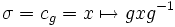# Quotient-pullbackable equals inner

Jump to: navigation, search
This article gives a proof/explanation of the equivalence of multiple definitions for the term inner automorphism
View a complete list of pages giving proofs of equivalence of definitions
This fact is related to: Extensible automorphisms problem
View other facts related to Extensible automorphisms problemView terms related to Extensible automorphisms problem |

## Statement

The following are equivalent for an automorphism$\sigma$ of a group$G$:

1. The automorphism is a quotient-pullbackable automorphism: For any homomorphism$\rho:H \to G$, there is an automorphism$\varphi$ of$H$,$\rho \circ \varphi = \sigma \circ \rho$.
2. The automorphism is an inner automorphism.

## Definitions used

### Quotient-pullbackable automorphism

An automorphism$\sigma$ of a group$G$ is termed quotient-pullbackable if given any surjective homomorphism$\rho: H \to G$ there is an automorphism$\varphi$ of$H$ such that$\rho \circ \varphi = \sigma \circ \rho$.

### Inner automorphism

Further information: Inner automorphism

An automorphism$\sigma$ of a group$G$ is termed an inner automorphism if there exists$g \in G$ such that$\sigma = c_g = x \mapsto gxg^{-1}$.